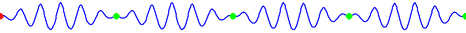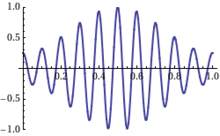# Phase velocityThis shows a wave with the group velocity and phase velocity going in different directions. The group velocity is positive, while the phase velocity is negative.

The phase velocity of a wave is the rate at which the phase of the wave propagates in space. This is the velocity at which the phase of any one frequency component of the wave travels. For such a component, any given phase of the wave (for example, the crest) will appear to travel at the phase velocity. The phase velocity is given in terms of the wavelength λ (lambda) and period T asEquivalently, in terms of the wave's angular frequency ω, which specifies angular change per unit of time, and wavenumber (or angular wave number) k, which represents the proportionality between the angular frequency ω and the linear speed (speed of propagation) νp,To understand where this equation comes from, consider a basic sine wave, A cos (kxωt). After time t, the source has produced ωt/2π = ft oscillations. After the same time, the initial wave front has propagated away from the source through space to the distance x to fit the same number of oscillations, kx = ωt.

Thus the propagation velocity v is v = x/t = ω/k. The wave propagates faster when higher frequency oscillations are distributed less densely in space. Formally, Φ = kxωt is the phase. Since ω = −dΦ/dt and k = +dΦ/dx, the wave velocity is v = dx/dt = ω/k.

## Relation to group velocity, refractive index and transmission speed

Since a pure sine wave cannot convey any information, some change in amplitude or frequency, known as modulation, is required. By combining two sines with slightly different frequencies and wavelengths,the amplitude becomes a sinusoid with phase speed Δωk. It is this modulation that represents the signal content. Since each amplitude envelope contains a group of internal waves, this speed is usually called the group velocity, vg.

In a given medium, the frequency is some function ω(k) of the wave number, so in general, the phase velocity vp = ω/k and the group velocity vg = dω/dk depend on the frequency and on the medium. The ratio between the speed of light c and the phase velocity vp is known as the refractive index, n = c/vp = ck/ω.

Taking the derivative of ω = ck/n with respect to k, yields the group velocity,Noting that c/n = vp, indicates that the group speed is equal to the phase speed only when the refractive index is a constant dn/dk = 0, and in this case the phase speed and group speed are independent of frequency, ω/k=dω/dk=c/n.

Otherwise, both the phase velocity and the group velocity vary with frequency, and the medium is called dispersive; the relation ω=ω(k) is known as the dispersion relation of the medium.

The phase velocity of electromagnetic radiation may – under certain circumstances (for example anomalous dispersion) – exceed the speed of light in a vacuum, but this does not indicate any superluminal information or energy transfer. It was theoretically described by physicists such as Arnold Sommerfeld and Léon Brillouin. See dispersion for a full discussion of wave velocities.

## References

### Footnotes

1. Nemirovsky, Jonathan; Rechtsman, Mikael C; Segev, Mordechai (9 April 2012). "Negative radiation pressure and negative effective refractive index via dielectric birefringence" (PDF). Optics Express. 20 (8): 8907–8914. Bibcode:2012OExpr..20.8907N. doi:10.1364/OE.20.008907. PMID 22513601.
2. "Phase, Group, and Signal Velocity". Mathpages.com. Retrieved 2011-07-24.

### Bibliography

• Crawford jr., Frank S. (1968). Waves (Berkeley Physics Course, Vol. 3), McGraw-Hill, ISBN 978-0070048607 Free online version
• Brillouin, Léon (1960), Wave Propagation And Group Velocity, New York and London: Academic Press Inc., ISBN 0-12-134968-3
• Main, Iain G. (1988), Vibrations and Waves in Physics (2nd ed.), New York: Cambridge University Press, pp. 214–216, ISBN 0-521-27846-5
• Tipler, Paul A.; Llewellyn, Ralph A. (2003), Modern Physics (4th ed.), New York: W. H. Freeman and Company, pp. 222–223, ISBN 0-7167-4345-0